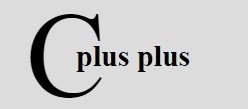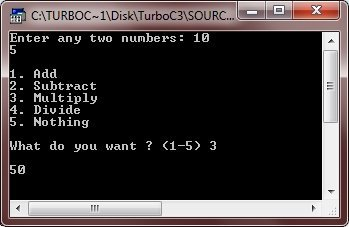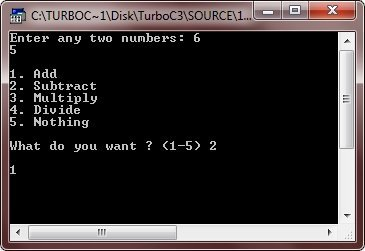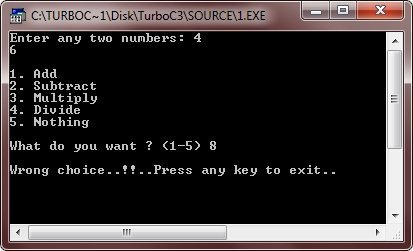# C++ Decision Making

Decision making statements in C++ allows you to specify one or more condition to be evaluated by your program to run specific statement(s). There are following types of decision making statements available in C++. You will learn all about these C++ decision making statements in detail, in C++ if if-else if-else-if switch Statements tutorial.

You will learn in detail about the above C++ decision making statements in next tutorial.

## C++ Decision Making Example

Let's take some example, demonstrating the above listed C++ decision making statements practically. As already told, that the explanation and details description of this chapter is described in the next tutorial.

```/* C++ Decision Making */

#include<iostream.h>
#include<conio.h>
#include<stdlib.h>
void main()
{
clrscr();
int a, b;
char choice;
cout<<"Enter any two numbers: ";
cin>>a>>b;
cout<<"\n";
cout<<"1. Add\n";
cout<<"2. Subtract\n";
cout<<"3. Multiply\n";
cout<<"4. Divide\n";
cout<<"5. Nothing\n";
cout<<"\nWhat do you want ? (1-5) ";
cin>>choice;
cout<<"\n";
switch(choice)
{
case '1' :
cout<<a+b;
break;
case '2' :
cout<<a-b;
break;
case '3' :
cout<<a*b;
break;
case '4' :
cout<<a/b;
break;
case '5' :
exit(1);
default :
cout<<"Wrong choice..!!..Press any key to exit..\n";
getch();
exit(2);
}
getch();
}```

Here is the sample run of the above C++ decision making program:Here is another sample run of the same, above C++ decision making program:Here is another C++ program, also demonstrating C++ decision making practically

```/* C++ Decision Making */

#include<iostream.h>
#include<conio.h>
#include<stdlib.h>
void main()
{
clrscr();
int a, b;
char choice;
cout<<"Enter any two numbers: ";
cin>>a>>b;
cout<<"\n";
cout<<"1. Add\n";
cout<<"2. Subtract\n";
cout<<"3. Multiply\n";
cout<<"4. Divide\n";
cout<<"5. Nothing\n";
cout<<"\nWhat do you want ? (1-5) ";
cin>>choice;
cout<<"\n";
if(choice == '1')
{
cout<<a+b;
}
else if(choice == '2')
{
cout<<a-b;
}
else if(choice=='3')
{
cout<<a*b;
}
else if(choice=='4')
{
cout<<a/b;
}
else if(choice=='5')
{
exit(1);
}
else
{
cout<<"Wrong choice..!!..Press any key to exit..\n";
getch();
exit(2);
}
getch();
}```

Here are the two sample runs of the above C++ decision making program:### More Examples

Here are some more C++ decision making programs, that you may like:

Tools
Calculator

Quick Links
Signup - Login - Give Online Test